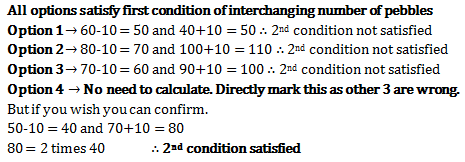# Problems on Numbers - Quantitative Aptitude (MCQ) questions for Q. 28908

Q.  Raman has 2 urns. Both these urns have some pebbles. If 20 pebbles from urn B are shifted to urn A then, the number of pebbles in each urn become interchanged. But if 10 pebbles from urn A are put into urn B, then number of pebbles in B are twice the number in A. How many pebbles do A and B have respectively?
- Published on 27 Mar 17

a. 60 and 40
b. 80 and 100
c. 70 and 90
d. 50 and 70